The SSM Procedure

Multivariate Cycle

The STATE statement option TYPE=CYCLE specifies a (2*dim)-dimensional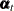, needed for defining a dim-dimensional cycle. As in the LL case, the first dim elements ofcorrespond to the needed dim-dimensional cycle, and the remaining dim elements contain some auxiliary quantities. The cycle model defined in this subsection requires a regular data type—that is, the CT option is not included. Let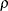denote the damping factor, and let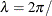period be the frequency associated with the cycle. The admissible parameter ranges are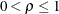and period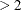, which implies that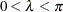. Let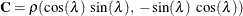, amatrix, and let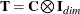, a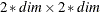matrix. With this notation, the transition equation associated withis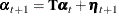where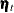is a sequence of zero mean, independent,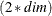-dimensional Gaussian vectors with covariance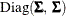. If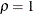, the initial condition is fully diffuse (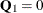and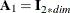). Otherwise, it is nondiffuse: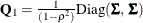and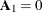.

The multivariate cycle is useful for capturing periodic behavior for multivariate time series data. The cycle term for the ith response variable is defined by a component that simply picks the ith element of. For example, the component cycle_i defined as follows can be used as a cycle term in the MODEL statement of the ith response variable:

state cycleState(dim) type=cycle  ...;
component cycle_2 = cycleState;